Skip to main content Accessibility help
Home

# Pinch-off of a viscous suspension thread

## Abstract

The pinch-off of a capillary thread is studied at large Ohnesorge number for non-Brownian, neutrally buoyant, mono-disperse, rigid, spherical particles suspended in a Newtonian liquid with viscosity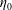$\unicode[STIX]{x1D702}_{0}$ and surface tension$\unicode[STIX]{x1D70E}$ . Reproducible pinch-off dynamics is obtained by letting a drop coalesce with a bath. The bridge shape and time evolution of the neck diameter,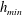$h_{\mathit{min}}$ , are studied for varied particle size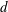$d$ , volume fraction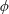$\unicode[STIX]{x1D719}$ and liquid contact angle$\unicode[STIX]{x1D703}$ . Two successive regimes are identified: (i) a first effective-viscous-fluid regime which only depends upon$\unicode[STIX]{x1D719}$ and (ii) a subsequent discrete regime, depending both on$d$ and$\unicode[STIX]{x1D719}$ , in which the thinning localises at the neck and accelerates continuously. In the first regime, the suspension behaves as an effective viscous fluid and the dynamics is solely characterised by the effective viscosity of the suspension,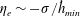$\unicode[STIX]{x1D702}_{e}\sim -\unicode[STIX]{x1D70E}/{\dot{h}}_{\mathit{min}}$ , which agrees closely with the steady shear viscosity measured in a conventional rheometer and diverges as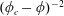$(\unicode[STIX]{x1D719}_{c}-\unicode[STIX]{x1D719})^{-2}$ at the same critical particle volume fraction,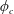$\unicode[STIX]{x1D719}_{c}$ . For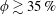$\unicode[STIX]{x1D719}\gtrsim 35\,\%$ , the thinning rate is found to increase by a factor of order one when the flow becomes purely extensional, suggesting non-Newtonian effects. The discrete regime is observed from a transition neck diameter,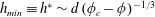$h_{\mathit{min}}\equiv h^{\ast }\sim d\,(\unicode[STIX]{x1D719}_{c}-\unicode[STIX]{x1D719})^{-1/3}$ , down to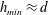$h_{\mathit{min}}\approx d$ , where the thinning rate recovers the value obtained for the pure interstitial fluid,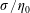$\unicode[STIX]{x1D70E}/\unicode[STIX]{x1D702}_{0}$ , and lasts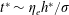$t^{\ast }\sim \unicode[STIX]{x1D702}_{e}h^{\ast }/\unicode[STIX]{x1D70E}$ .

## Corresponding author

Email address for correspondence: henri.lhuissier@univ-amu.fr

## References

Hide All
MathJax
MathJax is a JavaScript display engine for mathematics. For more information see http://www.mathjax.org.

## JFM classification

 Type Description Title VIDEO Movies Château et al. supplementary movie 1 Pinch-off of a capillary bridge of a suspension of particles with a diameter d = 10 micrometers and a particle volume fraction phi = 48%, suspended in pure PEG (close to 2500 times as viscous as water). In order to compensate for the continuously increasing rates of deformation at the bridge neck, the time in the movies is increasingly slowed down as pinching proceeds. The duration, t_0 - t, remaining before the pinch-off is indicated at the top. The width of the image is 4.45 mm.  Video (1.4 MB) Your browser does not support video. 1.4 MB VIDEO Movies Château et al. supplementary movie 2 Pinch-off of a capillary bridge of a suspension of particles with a diameter d = 135 micrometers and a particle volume fraction phi = 50%, suspended in pure PEG (close to 2500 times as viscous as water). In order to compensate for the continuously increasing rates of deformation at the bridge neck, the time in the movies is increasingly slowed down as pinching proceeds. The duration, t_0 - t, remaining before the pinch-off is indicated at the top. The width of the image is 4.45 mm.  Video (1.6 MB) Your browser does not support video. 1.6 MB

## Metrics

### Full text viewsFull text views reflects the number of PDF downloads, PDFs sent to Google Drive, Dropbox and Kindle and HTML full text views.

Total number of HTML views: 0
Total number of PDF views: 0 *Loading metrics...

### Abstract viewsAbstract views reflect the number of visits to the article landing page.

Total abstract views: 0 *Loading metrics...

* Views captured on Cambridge Core between <date>. This data will be updated every 24 hours.

Usage data cannot currently be displayed